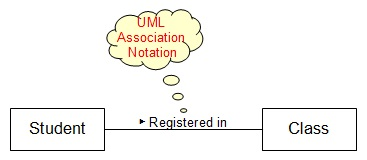Class Diagram - Association Notation

This section describes the Association Notation used in a UML Class Diagram. An Association Notation presents an association relation between two classes in which one class is related to the other class.

An Association Notation is a graphical notation used in a UML Class Diagram to represent an association relation between two classes in which one class is related to the other class.

An Association Notation is drawn as a solid line with two ends connected to the related classes. An Association Notation can also have a text label placed along the association line to describe the relation. A small solid triangle can be used to point away from the first class to the second class to imply that the label should be read as "<first class> <label> <second class>".

For example, the "Student" class and the "Class" class have an association that "A student is registered in a class". The association can be drawn as an Association Notation in a UML class diagram as shown below:UML Notation Shapes - Class Association

See next sections for other features of Association Notations.

Table of Contents